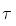Courses

# Notes | EduRev

## JEE : Notes | EduRev

The document Notes | EduRev is a part of the JEE Course JEE Revision Notes.
All you need of JEE at this link: JEE

Question 1: We can think of all nuclides as made up of a neutron-proton mixture that we can call nuclear matter. What is its density?
Solution:-
We know that this density is high because virtually all the mass of the atom is found in its tiny central nucleus. The volume of the nucleus (assumed spherical) of mass number A and radius R is
V = 4/3 πR3
= 4/3 π (R0A1/3)3
= 4/3 πR03A
Here we have used Eq. R=R0A1/3 to obtain the third expression. Such a nucleus contains A nucleons so that its nucleon number density ρn, expressed in nucleons per unit volume, is
ρn = A / V
= [A] / [(4/3)(πR03A)]
= 3/[(4π) (1.2 fm)3]
= 0.138 nucleon/fm3.
We can consider nuclear matter as having a single density for all nuclides only because A cancels in the equation above.
The mass of a nucleon (neutron or proton) is about 1.67 x 10-27 kg. The mass density of nuclear matter in SI units is then
ρ = (0.138 nucleon/fm3) (1.67×10-27 kg/nucleon) × (1015 fm/m)3
≈ 2×1017 kg/m3
Thus from the above observation, we conclude that, its density would be 2×1017 kg/m3 . This is about 2×1014 times the density of water.

Question 2:
(a) How much energy is required to separate the typical middle-mass nucleus 120Sn into its constituent nucleons?
(b) What is the binding energy per nucleon for this nuclide?
Solution:-
(a) We can find this energy from Q = Δmc2. Following standard particle, we carry out such calculations in terms of the masses of the neutral atoms involved, not those of the bare nuclei. One atom of  120Sn (nucleus plus 50 electrons) has a mass of 119.902 199 u. This atom can be separated into 50 hydrogen atoms (50 protons, each with one of the 50 electrons) and 70 neutrons. Each hydrogen atom has a mass of 1.007825 u, and each neutron a mass of 1.008665 u. The combined mass of the constituent particle is
m = (50×1.007825 u) + (70×1.008665 u)
= 120.997 80 u
This exceeds the atomic mass of 120Sn by
Δm = 120.99780 u – 119.902 199 u
= 1.095601 u
≈ 1.096 u
Note that since the masses of the 50 electrons cancel in the subtraction, this is also the mass difference that is obtained when a bare 120Sn nucleus is separated into 50 (bare) protons and 70 neutrons. In energy terms this mass difference becomes
Q = Δmc2
= (1.096 u) (931.5 MeV/u)
= 1021 MeV
Therefore, the energy is required to separate the typical middle-mass nucleus 120Sn into its constituent nucleons would be 1021 MeV.
(b) The total binding energy Q is the total energy that must be supplied to dismantle the nucleus. The binding energy per nucleon En is then
En = Q/A = 1021 MeV/120 = 8.51 MeV/nucleon
From the above observation we conclude that, the binding energy per nucleon for this nuclide would be 8.51 MeV/nucleon.

Question 3: Calculate the disintegration energy Q for the beta decay of 32P, as described by equation 32P→32S + e- + ν  (= 14.3 d). The needed atomic masses are 31.97391 u for 32P and 31.97207 u for 32S.
Solution:-
Because of the presence of the emitted electron, we must be careful to distinguish between nuclear and atomic masses. Let the boldface symbols mp and ms represent the nuclear masses 32P and 32S and let the italic symbols mp and ms represent their atomic masses. We take the disintegration energy Q to be Δmc2, where, from Eq. 32P→32S + e- + ν  (= 14.3 d),
Δm = mp – (ms + me),
in which me is the mass of the electron. If we add and subtract 15me on the right side of this equation, we obtain
Δm = (mp + 15me) – (m+ 16me)
The quantities in parentheses are the atomic masses in this way, the mass of the emitted electron is automatically taken into account. (This will not work for positron emission.)
The disintegration energy for the  32P decay is then
Q = Δmc2
= (31.97391 u – 31.97207 u) (931.5 MeV/u)
= 1.71 MeV
Therefore, from the above observation we conclude that, the disintegration energy Q for the beta decay of 32P would be 1.71 MeV.

Offer running on EduRev: Apply code STAYHOME200 to get INR 200 off on our premium plan EduRev Infinity!

233 docs

,

,

,

,

,

,

,

,

,

,

,

,

,

,

,

,

,

,

,

,

,

;